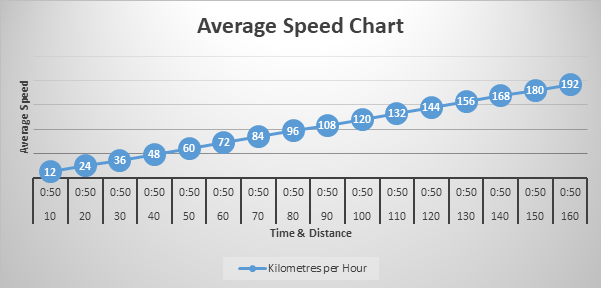## Average Speed Calculator Tool

• Home
• Average Speed Calculator Tool

## Average Speed Calculator Tool

Calculates the Average Speed based on time and distance

This tool will help you calculate the average speed based on the time and distance of the service. Don’t waste time trying to work out the formula, enter the distance of the trip and the time it has or will take, and we will show you the applicable average speed.

This tool can help in various ways, whether you are calculating the average speed:

P    of a walking in route,

P    for a cycling trip,

P    to validate the accuracy of time and distance date,

P    of a bus service,

P    it takes to travel on a train,

P    a plane travel’s at, or

The best thing about our Average Speed Calculator is it free.

Average Speed Formula

Average Speed = Total Distance / Total Time

The chart below shows the average speed using various distances between 10 and 160 while the journey remains constant at 50 minutes.